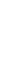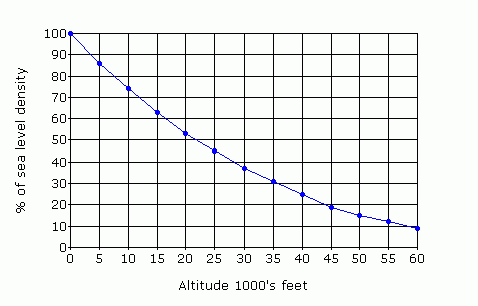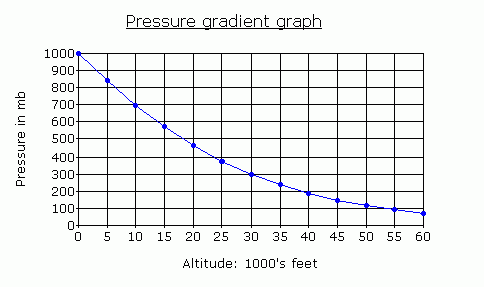weight of atmosphere

Atmospheric pressure reflects the average density and thus the weight of the column of air above a given level, thus the pressure at a point on the earth's surface must be greater than the pressure at any height above it. An increase in surface pressure denotes an increase in mass, not thickness, of the column of air above the surface point. Similarly a decrease in surface pressure denotes a decrease in the mass. The gradient is the difference in pressure vertically, and horizontally.

The air throughout the column is compressed by the weight of the atmosphere above it and thus the density of a column of air is greatest at the surface and decreases exponentially with altitude as shown in the following graph which is a plot of the rate of decrease in density with increase in altitude. The plot is for dry air at mid-latitudes. ( Mid latitudes are usually accepted to be the areas between the 30° and 60° lines while low latitudes lie between the equator and 30° and high latitudes between 60° and the pole.) The atmosphere at about 22 000 feet has only 50% of the sea level density, density decreases by about 3% per 1000 feet between sea level and 18 500 feet and thereafter the density lapse rate slows.The dry air density gradient in mid-latitudes, refer table in 2.1

As the pressure decreases with height so, in any parcel of air, the downwards pressure over the top of the parcel must be less than the upwards pressure under the bottom: thus within the parcel there is a vertical component of the pressure gradient force acting upward; generally this force is balanced by the gravitational force so the net sum of forces is zero and the parcel floats in equilibrium. This balance of forces is called the hydrostatic balance. When the two do not quite balance the difference is the buoyancy force which is the upward, or downward, force exerted on a parcel of air arising from the density difference between the parcel and the surrounding air.

Atmospheric pressure also varies horizontally, due to air mass changes associated with the regional thickness of the atmospheric layer. The resultant horizontal pressure gradient force, not being balanced by gravity, forces air to move from regions of higher pressure towards regions of lower pressure but the movement is modified by Coriolis effect. The horizontal force is about 1/15 000 of the vertical component.

(Advection is the term used for the transport of momentum, heat, moisture, vorticity or other atmospheric properties, by the horizontal movement of air)

The following graph plots the average mid-latitude vertical pressure gradient and shows how the overall vertical decrease in pressure – the pressure lapse rate – slows exponentially as the air becomes less dense with height. In a denser, or colder, air mass the pressure reduces at a faster rate, conversely in less dense, or warmer, air the pressure reduces at a slower rate. (The hydrostatic equation states that the vertical change in pressure, between two levels in any column of air, is equal to the weight, per unit area, of the air in the column.) If two air columns have the same pressure change from top to bottom the denser column will be shorter, conversely if the two columns have the same height the denser column will have a larger change in pressure from top to bottom.In the ICAO standard atmosphere, details of which are shown in section 2.1, the rate of altitude change for each 1 hPa change in pressure is approximately:

 zero to 5000 feet: 30 feet/hPa or 34 hPa per 1000 feet 5000 to 10 000 feet: 34 feet/hPa or 29 hPa per 1000 feet 10 000 to 20 000 feet: 43 feet/hPa or 23 hPa per 1000 feet 20 000 to 40 000 feet: 72 feet/hPa or 14 hPa per 1000 feet

The change in altitude for one hectopascal change in pressure can be roughly calculated from the absolute temperature and the pressure at the level using the equation: = 96T/P feet.Your browser does not support inline frames or is currently configured not to display inline frames.